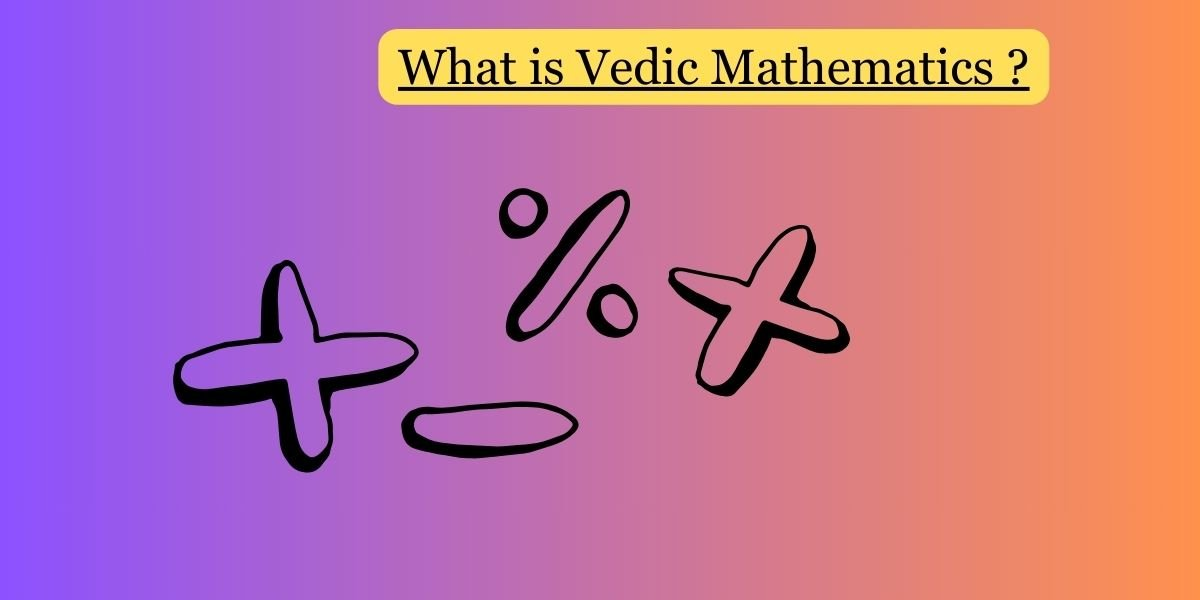Vedic Mathematics is a system of mathematical techniques that originated in ancient India. The term “Vedic” refers to the Vedas, which are a set of ancient sacred texts in Hinduism. Vedic Mathematics was first introduced by Swami Bharati Krishna Tirtha in the early 20th century in his book “Vedic Mathematics,” where he compiled and presented various mathematical techniques found in the Vedas.

The main features of Vedic Mathematics include:

1. Sutras (Formulas): Vedic Mathematics is based on a set of 16 Sutras, which are short aphorisms or formulas that cover various mathematical operations. These Sutras serve as guiding principles for solving mathematical problems.
2. Flexibility and Efficiency: The techniques in Vedic Mathematics are known for their flexibility and efficiency. They provide alternative and often quicker methods for arithmetic and algebraic calculations compared to conventional methods.
3. Mental Math: Vedic Mathematics emphasizes mental calculation techniques, enabling individuals to perform complex mathematical operations in their minds. This is particularly useful for quick and accurate calculations.
4. Applicability to Various Fields: The techniques in Vedic Mathematics are not limited to specific mathematical operations but can be applied to a wide range of mathematical problems, including multiplication, division, algebra, calculus, and more.
5. Cross-checking Methods: Many Vedic Math techniques come with built-in cross-checking methods. This means that the correctness of a calculation can often be verified by using a complementary method, providing a level of self-verification.
6. Simplified Rules for Special Cases: Vedic Mathematics provides simplified rules for specific cases, such as squaring numbers, multiplying numbers close to a base, or finding square roots.

It’s important to note that while Vedic Mathematics has gained popularity and is taught in some educational institutions, it is not universally accepted as a mainstream mathematical approach. Some critics argue that the efficiency of Vedic Mathematics techniques may be subjective and that conventional methods are equally powerful.

Whether one chooses to use Vedic Mathematics or traditional methods depends on personal preferences and the specific requirements of the mathematical problem at hand. Many people find Vedic Mathematics techniques interesting and enjoyable as they offer a unique perspective on mathematical calculations.